International
Tables for
Crystallography
Volume D
Physical properties of crystals
Edited by A. Authier

International Tables for Crystallography (2013). Vol. D, ch. 1.11, pp. 275-276

## Section 1.11.6.1. Tensor atomic factors: internal symmetry

V. E. Dmitrienko,a* A. Kirfelb and E. N. Ovchinnikovac

aA. V. Shubnikov Institute of Crystallography, Leninsky pr. 59, Moscow 119333, Russia,bSteinmann Institut der Universität Bonn, Poppelsdorfer Schloss, Bonn, D-53115, Germany, and cFaculty of Physics, M. V. Lomonosov Moscow State University, Leninskie Gory, Moscow 119991, Russia
Correspondence e-mail:  dmitrien@crys.ras.ru

#### 1.11.6.1. Tensor atomic factors: internal symmetry

| top | pdf |

Different types of tensors transform under the action of the extended orthogonal group (Sirotin & Shaskolskaya, 1982) aswhere the coefficients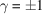depend on the kind of tensor (see Table 1.11.6.1) andare coefficients describing proper rotations.

 Table 1.11.6.1| top | pdf | Coefficientscorresponding to various kinds of tensor symmetry with respect to space inversion, rotations, and time reversal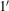Tensor typeExampleTransformation type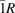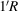Even Strain 1 1 1 1
Electric Electric field 1 −1 1 −1
Magnetic Magnetic field 1 1 −1 −1
Magnetoelectric Toroidal moment 1 −1 −1 1

Various parts of the resonant scattering factor (1.11.6.3)possess different kinds of symmetry with respect to: (1) space inversionor parity, (2) rotationsand (3) time reversal. Both dipole–dipole and quadrupole–quadrupole terms are parity-even, whereas the dipole–quadrupole term is parity-odd. Thus, dipole–quadrupole events can exist only for atoms at positions without inversion symmetry.

It is convenient to separate the time-reversible and time-non-reversible terms in the contributions to the atomic tensor factor (1.11.6.3). The dipole–dipole contribution to the resonant atomic factor can be represented as a sum of an isotropic, a symmetric and an antisymmetric part, written as (Blume, 1994)where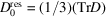,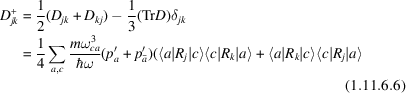and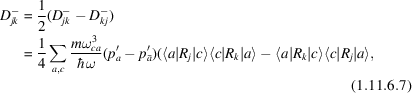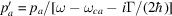and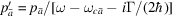;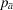means the probability of the time-reversed state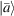. If, for example,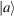has a magnetic quantum number m, thenhas a magnetic quantum number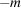.

In non-magnetic crystals, the probability of states with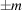is the same, so that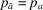and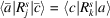; in this case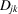is symmetric under permutation of the the indices.

Similarly, the dipole–quadrupole atomic factor can be represented as (Blume, 1994)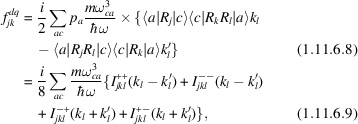where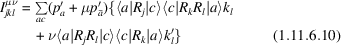with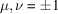. In (1.11.6.10)the first plus (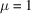) corresponds to the non-magnetic case (time reversal) and the minus (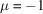) corresponds to the time-non-reversal magnetic term, while the secondcorresponds to the symmetric and antisymmetric parts of the atomic factor. We see thatcan contribute only to scattering, while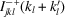can contribute to both resonant scattering and resonant X-ray propagation. The latter term is a source of the so-called magnetochiral dichroism, first observed in Cr2O3 (Goulon et al., 2002, 2003), and it can be associated with a toroidal moment in a medium possessing magnetoelectric properties. The symmetry properties of magnetoelectic tensors are described well by Sirotin & Shaskolskaya (1982), Nye (1985) and Cracknell (1975). Which magnetoelectric properties can be studied using X-ray scattering are widely discussed by Marri & Carra (2004), Matsubara et al. (2005), Arima et al. (2005) and Lovesey et al. (2007).

It follows from (1.11.6.8)and (1.11.6.10)that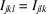and the dipole–quadrupole term can be represented as a sum of the symmetric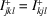and antisymmetric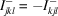parts. From the physical point of view, it is useful to separate the dipole–quadrupole term intoand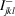, because onlyworks in conventional optics where. The dipole–quadrupole terms are due to the hybridization of excited electronic states with different spacial parities, i.e. only for atomic sites without an inversion centre.

The pure quadrupole–quadrupole term in the tensor atomic factor is equal to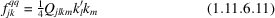with the fourth-rank tensorgiven by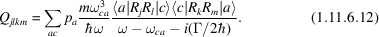This fourth-rank tensorhas the following symmetries: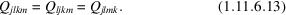We can define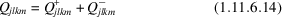with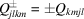, where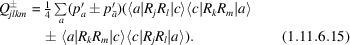We see thatvanishes in time-reversal invariant systems, which is true for non-magnetic structures.

### References

Cracknell, A. P. (1975). Group Theory in Solid-State Physics. London: Taylor and Francis Ltd.Google Scholar
Arima, T., Jung, J.-H., Matsubara, M., Kubota, M., He, J.-P., Kaneko, Y. & Tokura, Y. (2005). Resonant magnetochiral X-ray scattering in LaFeO3: observation of ordering of toroidal moments. J. Phys. Soc. Jpn, 74, 1419–1422.Google Scholar
Blume, M. (1994). Resonant X-ray diffraction and polarization analysis at the iron K-edge. In Resonant Anomalous X-ray Scattering. Theory and Applications, edited by G. Materlik, C. J. Sparks & K. Fischer, pp. 91–97. Amsterdam: North-Holland.Google Scholar
Goulon, J., Rogalev, A., Wilhelm, F., Goulon-Ginet, C. & Carra, P. (2002). X-ray magnetochiral dichroism: a new spectroscopic probe of parity nonconserving magnetic solids. Phys. Rev. Lett. 88, 237401.Google Scholar
Goulon, J., Rogalev, A., Wilhelm, F., Goulon-Ginet, C., Carra, P., Marri, I. & Brouder, C. (2003). X-ray optical activity: application of sum rules. J. Exp. Theor. Phys. 97, 402–431.Google Scholar
Lovesey, S. W., Fernández-Rodríguez, J., Blanco, J. A., Sivia, D. S., Knight, K. S. & Paolasini, L. (2007). Vanadium magnetoelectric multipoles in V2O3. Phys. Rev. B, 75, 014409.Google Scholar
Marri, I. & Carra, P. (2004). Scattering operators for E1–E2 X-ray resonant diffraction. Phys. Rev. B, 69, 113101.Google Scholar
Matsubara, M., Shimada, Y., Arima, T., Taguchi, Y. & Tokura, Y. (2005). Observation of nonreciprocal magnetoelectric X-ray scattering in magnetite. Phys. Rev. B, 72, 220404.Google Scholar
Nye, J. F. (1985). Physical Properties of Crystals: Their Representation by Tensors and Matrices. Oxford University Press.Google Scholar
Sirotin, Y. & Shaskolskaya, M. P. (1982). Fundamentals of Crystal Physics. Moscow: Mir.Google Scholar